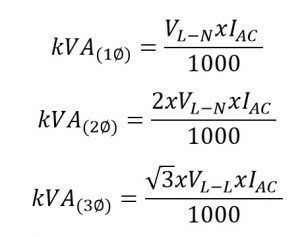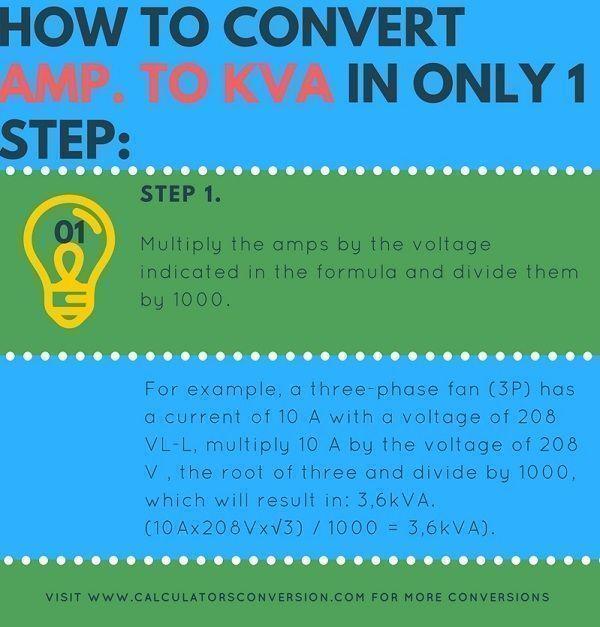# Amp to kVA conversion – calculations for transformer and generator free.

With this calculator you can convert from Amps. to kVA or kVA to Amps easily, quickly and free any electric power.

For greater ease we explain which formula is used for the calculation, how to calculate from Amp. to kVA in 1 single step , several examples and a table with the main Amps to kVA conversions.

## Amp. to kVA calculation formula for generator and transformer, AC, 3 phase, 2 phase, 1 phase:• IAC=Ampere.
• VL-L=Volts Line-Line.
• VL-N=Volts Line-Neutral.
• IAC1Ø=Current/Ampere 1 phase.
• IAC2Ø=Current/Ampere 2 phase.
• IAC3Ø=Current/Ampere 3 phase.

## How to convert Amp. to kVA in only 1 step:### Step 1:

Multiply the amps by the voltage indicated in the formula and divide them by 1000. For example, a three-phase fan (3P) has a current of 10 A with a voltage of 208 VL-L, multiply 10 A by the voltage of 208 V , the root of three and divide by 1000, which will result in: 3,6kVA. (10Ax208Vx√3) / 1000 = 3,6kVA).

## Examples of Amps to kVA conversions:

Example 1:

A monophasic hair dryer has an amperage of 2 Amp and a voltage of 110V, how much power in kVA will the dryer have ?.

Answer: // The only thing to do is multiply the Amps by the voltage and the result divide it by 1000, in the following way: (2Ampx110V) / 1000 = 0.22kVA.

Example 2:

A three-phase industrial juice extractor has an amperage of 1, amperes and a voltage of 208V (Volts LL), how many kVA will the extractor have?

Answer: // To know the result, you must multiply the voltage by the amperage and by the root of three, and then divide the result by 1000, in the following way: (1.4Ampx208Vx√3) / 1000 = 0.5kVA

Example 3:

A three-phase washing machine for a hotel, has a 4Amp amperage and a voltage of 220Volts (Volts Linea-Linea), how many kVA will the washing machine have?

Answer: // As in the previous examples we must multiply 4 amperex220Voltsx√3, the result of this multiplication should be divided by 1000, for a result of: 1,52kVA

## Amp. to kVA, table for conversion, equivalence, transformation (Voltage = 220, AC, 3F):

 How many Amps are: Equivalence in kVA 10 Amps Equivalent to 3.81 kVA 20 Amps 7.62 kVA 30 Amps 11.43 kVA 40 Amps 15.24 kVA 50 Amps 19.05 kVA 60 Amps 22.86 kVA 70 Amps 26.67 kVA 80 Amps 30.48 kVA 90 Amps 34.29 kVA 100 Amps 38.11 kVA 200 Amps 76.21 kVA 300 Amps 114.32 kVA 400 Amps 152.42 kVA 500 Amps 190.53 kVA 600 Amps 228.63 kVA 700 Amps 266.74 kVA 800 Amps 304.84 kVA 900 Amps 342.95 kVA 1000 Amps 381.05 kVA 2000 Amps 762.10 kVA 3000 Amperes 1143.15 kVA 4000 Amps 1524.20 kVA 5000 Amps 1905.26 kVA

Note : The conversions of the previous table were made taking into account a voltage of 220V, with a three-phase AC power. For different variables you should use the calculator that appears at the beginning.

Spanish version Amps. to kVA

Rate this calculator Amps to kVA:  [kkstarratings]